# List of formulas related to direct Current

## Electric Current

I: current (A), q: charge (C), t: time

$$I = {\frac{ \Delta q}{ \Delta t}}(A)$$

### Ohm's Law

U: electric potential difference between the ends of the resistor R

$$I = {\frac{U}{R}}$$

### Work Created By A Current (Joule's Law)

$$W = U.I.t = I^2 R$$

### Electrical Power

$$P = U.I = I^2 R$$

### Resistance

ρ: resistivity of the material (Ωm), l: length (m), A: cross-section area (a2)

$$R = \rho \frac{l}{A}$$ $$R = \rho \frac{U}{l}(\Omega)$$

### Series Circuits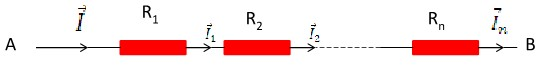UAB = U1= U2 = .... = Un

IAB = I1= I2 = .... = In

RAB = R1= R2 = .... = Rn

### Parallel Circuits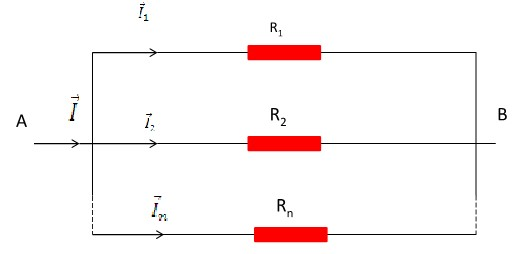$$U_{AB} = U_1 = U_2 = .... = U_n$$ $$I_{AB} = I_1 = I_2 = .... = I_n$$ $$\frac{1}{R_{AB}} = \frac{1}{R_1} = \frac{1}{R_2} = .... = \frac{1}{R_n}$$

### Work Or Heat Energy Produced By A Resistor

$$W = IR^2 t = \frac{U^2}{R} t$$

### Power Consumption Of A Resistor

$$Q = IR^2 = \frac{U^2}{R}$$

### Electromotive Force Of An Electrical Generator

W: work of force (J), q: charge(c)

$$\zeta = \frac{W}{q}(V)$$

### Generators In Series

r: internal resistance of the generator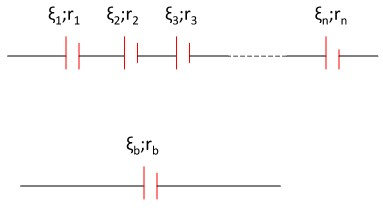$$\zeta _b = \zeta _1 + \zeta _2 + .... + \zeta _n$$ $$r _b = r _1 = r _2 = .... = r _n$$ $$(\zeta _1 : r _1) = (\zeta _2 : r _2) = .... = (\zeta _n : r _n)$$ $$\zeta _b = n\zeta$$ $$r_b = n.r$$

### Generators In Parallel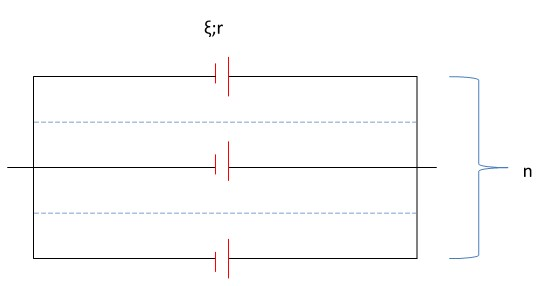$$r_b = \frac{r}{n}$$ $$\zeta _b = \zeta$$

### Generators In Series-Parallel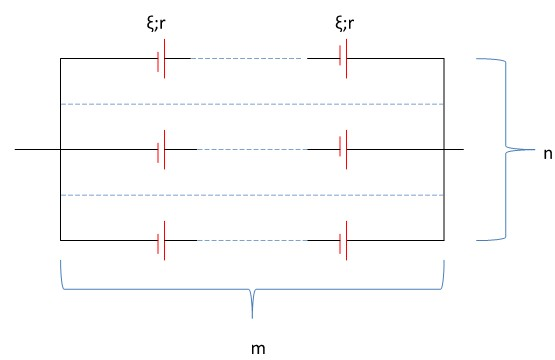$$\zeta _b = m\zeta$$ $$r_b = \frac{m}{n} r$$

### Work And Power Of Generator

H: Efficiency of genarator

$$W _g = \zeta.I.t$$ $$P _g = \frac{W_g}{t} = \zeta.I$$ $$H = \frac{U}{\zeta} = \frac{R}{R + r}$$